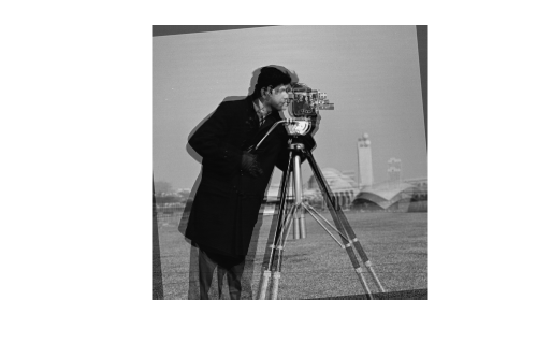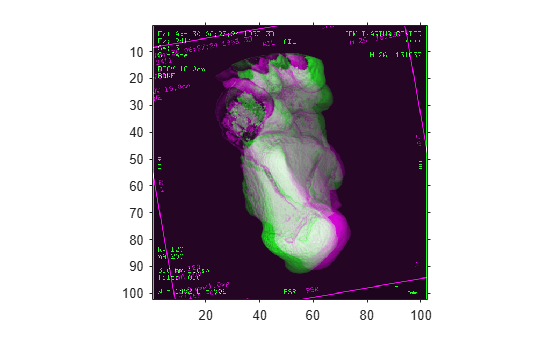# imshowpair

Compare differences between images

## Syntax

``obj = imshowpair(A,B)``
``obj = imshowpair(A,RA,B,RB)``
``obj = imshowpair(___,method)``
``obj = imshowpair(___,Name,Value)``

## Description

example

````obj = imshowpair(A,B)` creates a composite RGB image showing `A` and `B` overlaid in different color bands. To choose another type of visualization of the two images, use the `method` argument. If `A` and `B` are different sizes, `imshowpair` pads the smaller dimensions with zeros on the bottom and right edges so that the two images are the same size. By default, `imshowpair` scales the intensity values of `A` and `B` independently from each other. `imshowpair` returns `obj`, an image object.```

example

````obj = imshowpair(A,RA,B,RB)` displays the differences between images `A` and `B`, using the spatial referencing information provided in `RA` and `RB`. `RA` and `RB` are spatial referencing objects.```

example

````obj = imshowpair(___,method)` uses the visualization method specified by `method`.```

example

````obj = imshowpair(___,Name,Value)` specifies additional options with one or more `Name,Value` pair arguments, using any of the previous syntaxes.```

## Examples

collapse all

Display a pair of grayscale images with two different visualization methods, `'diff'` and `'blend'`.

Load an image into the workspace. Create a copy with a rotation offset applied.

```A = imread('cameraman.tif'); B = imrotate(A,5,'bicubic','crop');```

Display the difference of `A` and `B`.

`imshowpair(A,B,'diff')`Display a blended overlay of `A` and `B`.

```figure imshowpair(A,B,'blend','Scaling','joint')```Read an image. Create a copy and apply rotation and a brightness adjustment.

```A = dicomread('CT-MONO2-16-ankle.dcm'); B = imrotate(A,10,'bicubic','crop'); B = B * 0.2;```

In this example, we know that the resolution of images `A` and `B` is 0.2mm. Provide this information using two spatial referencing objects.

```RA = imref2d(size(A),0.2,0.2); RB = imref2d(size(B),0.2,0.2);```

Display the images with the default method (`'falsecolor'`) and apply brightness scaling independently to each image. Specify the axes that will be the parent of the image object created by `imshowpair`.

```figure; hAx = axes; imshowpair(A,RA,B,RB,'Scaling','independent','Parent',hAx);```## Input Arguments

collapse all

Image to be displayed, specified as a grayscale, truecolor, or binary image.

Image to be displayed, specified as a grayscale, truecolor, or binary image.

Spatial referencing information about an input image, specified as spatial referencing object, of class `imref2d`.

Spatial referencing information about an input image, specified as spatial referencing object, of class `imref2d`.

Visualization method to display combined images, specified as one of the following values.

ValueDescription
`'falsecolor'`Creates a composite RGB image showing `A` and `B` overlaid in different color bands. Gray regions in the composite image show where the two images have the same intensities. Magenta and green regions show where the intensities are different. This is the default method.
`'blend'`Overlays `A` and `B` using alpha blending.
`'checkerboard'`Creates an image with alternating rectangular regions from `A` and `B`.
`'diff'`Creates a difference image from `A` and `B`.
`'montage'`Places `A` and `B` next to each other in the same image.

Data Types: `char` | `string`

### Name-Value Arguments

Specify optional pairs of arguments as `Name1=Value1,...,NameN=ValueN`, where `Name` is the argument name and `Value` is the corresponding value. Name-value arguments must appear after other arguments, but the order of the pairs does not matter.

Before R2021a, use commas to separate each name and value, and enclose `Name` in quotes.

Example: `'Scaling','joint'` scales the intensity values of `A` and `B` together as a single data set.

Output color channel for each input image, specified as one of the following values:

 `[R G B]` A three element vector that specifies which image to assign to the red, green, and blue channels. The R, G, and B values must be 1 (for the first input image), 2 (for the second input image), and 0 (for neither image). `'red-cyan'` A shortcut for the vector `[1 2 2]`, which is suitable for red/cyan stereo anaglyphs. `'green-magenta'` A shortcut for the vector `[2 1 2]`, which is a high contrast option, ideal for people with many kinds of color blindness.

Interpolation technique used when scaling an image, specified as the comma-separated pair consisting of `'Interpolation'` and one of the following values.

ValueDescription
`'nearest'`Nearest neighbor interpolation (default)
`'bilinear'`Bilinear interpolation

Parent of image object created by `imshowpair`, specified as an axes object.

Intensity scaling option, specified as one of the following values.

 `'independent'` Scales the intensity values of `A` and `B` independently from each other. `'joint'` Scales the intensity values in the images jointly as if they were together in the same image. This option is useful when you want to visualize registrations of monomodal images, where one image contains fill values that are outside the dynamic range of the other image. `'none'` No additional scaling.

Data Types: `char` | `string`

## Output Arguments

collapse all

Visualization of two images, returned as an image object.

## Tips

• Use `imfuse` to create composite visualizations that you can save to a file. Use `imshowpair` to display composite visualizations to the screen.

## Version History

Introduced in R2012a